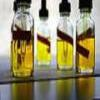#### You may also like### Mathematical Issues for Chemists

A brief outline of the mathematical issues faced by chemistry students.### Reaction Rates

Explore the possibilities for reaction rates versus concentrations with this non-linear differential equation### Catalyse That!

Can you work out how to produce the right amount of chemical in a temperature-dependent reaction?

# Mixing pH

##### Age 16 to 18 Challenge Level:

1) The initial equation can be rearranged to give: $$[H^+] = 10^{-pH}$$

The pH of water is 7, and so: $$[H^+] = 10^{-7}mol/l.$$

Thus in 1l of water there are 10$^{-7}$ moles of hydrogen ions.

2) If the pH is 2, then $[H^+] = 10^{-2} mol/l$

If this is diluted to a volume of 2 litres from the initial 1 litre, then $[H^+] = 0.5 \times 10^{-2}mol/l$.
$$\therefore pH = -log_{10}(0.5 \times 10^{-2}) = 2.30\ (2\ d.p)$$

3) If pH = 1.3, then $[H^+] = 10^{-1.3} mol/l$

Therefore, in 100ml (= 0.1l), there are $0.1 \times 10 ^{-1.3}$ moles.

We want to dilute this such that the pH = 2, which is the same as $[H^+] = 10^{-2} mol/l$.

$$\therefore \frac{0.1 \times 10^{-1.3}}{Vol} = 10^{-2}$$
$$Vol = 0.501l$$

Therefore, since we have already 100ml of solution, this requires the addition of 401ml.

4) For the first acid, pH = 3, therefore $[H^+] = 10^{-3} mol/l$.
Since there is 400ml of this acid, then the number of $H^+$ is $0.4 \times 10^{-3}$ moles

For the second acid, pH = 4, therefore $[H^+] = 10^{-4} mol/l$.
Since there is 300ml of this acid, the number of $H^+$ is $0.3 \times 10^{-4}$ moles

If these are mixed, there are a total of $4.3 \times 10^{-4}$ moles of $H^+$ in a volume of 0.7l. Therefore, $$[H^+] = 6.14 \times 10^{-4} mol/l$$

$$\therefore pH = -log_{10}[H^+] = 3.21\ (2\ d.p.)$$